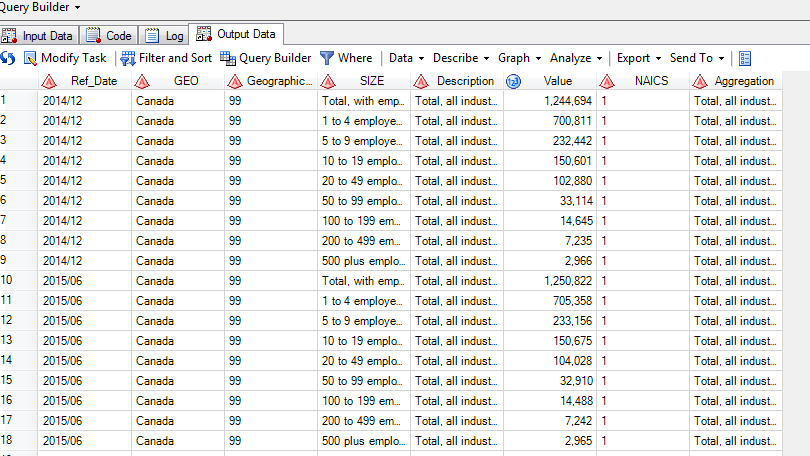## Linear interpolation in SAS

I have a SAS dataset of business population counts in Canada that was published by Statistics Canada. The data are published semi-annually. I currently have data for each of Dec. 2014 and June 2015 for business population counts by NAICS industry by employee size. However I would like to generate monthly counts by month between Dec. 2014 to June 2015. I have attached a picture of the data set. How would I code this in SAS? Thanks.11 REPLIES 11

## Re: Linear interpolation in SAS

I guess, that PROC EXPAND could solve it

## Re: Linear interpolation in SAS

Do you want to just roll up the totals to the month level?  Try this:

``````proc sql;
create table work.output as
select t1.ref_date,
t1.size,
/* totalcount */
(sum(t1.value)) format=best10. as totalcount
from work.data t1
group by t1.ref_date,
t1.size;
quit;
``````

But note that your date value is currently a CHAR -- not a SAS date.  If you want to perform any sort of time series analysis you'll want to convert that to a date.  You can convert it "on the fly" in SQL with an INPUT function and the ANYDTDTM informat.

``````proc sql;
create table work.output as
select datepart(input(t1.ref_date,anydtdtm7.)) as Month format=yymmd7.,
t1.size,
/* totalcount */
(sum(t1.value)) format=best10. as totalcount
from work.data t1
group by t1.ref_date,
t1.size;
quit;
``````

## Re: Linear interpolation in SAS

Thanks for the reply Chris.  I want to go to a higher frequency -- from semi-annual to monthly in my dataset.  Currently I have observations for Dec. 2014 and June 2015 as shown.  So I want to generate values by month (via linear interpolation).  How would you modify the code you provided?

Thanks again...Jack

## Re: Linear interpolation in SAS

Oh, I see -- I misunderstood, thinking you already had monthly.  Okay...

You still need to convert your ref_date to a date (number) value, so this piece still applies.

`datepart(input(t1.ref_date,anydtdtm7.)) as Month format=yymmd7.,`

Then as @Klara said, PROC EXPAND can help.  It's part of SAS/ETS.  If using SAS Enterprise Guide, check out Tasks->Time Series->Prepare Time Series Data.  If you don't have SAS/ETS, you might have to look up a data step implementation of a similar interpolation in a SAS Global Forum paper.  If you have just two categorical months, interpolation at the month level might be tricky to do with any reliable trending values.

## Re: Linear interpolation in SAS

Thanks Chris....I will give this a try and let you know how it goes...

Jack

## Re: Linear interpolation in SAS

Thanks for the help Chirs.  In SAS EG I tried going the route you mention below but I got a message that informed me that I need SAS EG on my machine in order for this feature to work.  I do not have ETS right now.  Thanks for the input...JackKsharp
Super User

## Re: Linear interpolation in SAS

```If you are using IML . Check Rick's blog:

http://blogs.sas.com/content/iml/2012/03/16/linear-interpolation-in-sas.html

```Ksharp
Super User

## Re: Linear interpolation in SAS

```Best choice is PROC EXPAND if you have ETS. OR try this code:

data have;
input x;
cards;
.
12
.
.
24
25
.
.
2
.
;
run;
data want;
retain y1 y2;

do i=1 by 1 until(not missing(x) or last1);
set have end=last1;
end;
y1=x;

do j=1 by 1 until(not missing(x) or last2);
set have end=last2;
if missing(x) then new_x=y2+j*(y1-y2)/i;
else new_x=x;
output;
end;
y2=x;

drop y1 y2 i j;
run;

data want;
set want;
n+1;
group=ifc(missing(x),'Interp','Raw');
run;
proc sgplot data=want;
series x=n y=new_x;
scatter x=n y=new_x/group=group markerattrs=(symbol=starfilled);
run;
run;

```

## Re: Linear interpolation in SAS

Hi Xia, Thanks for the reply. The data I have is semi-annual data (Dec. 2014 and June 2015 periods only) on business population counts by region from Statistics Canada. I want expand the series to show the monthly values between these two months (Jan. 2015, Feb. 2015, etc.). So the code would need to replicate the months between these end dates. Plus I want to include the remaining fields as shown in the screen shot. How can I use your code to generate this expanded data set? Thanks JackKsharp
Super User

## Re: Linear interpolation in SAS

Post data ,Not picture, Noone would like to type it for you .

``````
data have;
input date : anydtdte. size \$ value;
format date yymms.;
cards;
2014/12 A 124
2014/12 B 70
2015/06 A 140
2015/06 B 80
;
run;
proc sort data=have;by size date;run;
data temp;
merge have have(firstobs=2 keep=date size rename=(date=_date size=_size));
output;
if size=_size then do;
start=date;
do i=1 to intck('month',date,_date)-1;
date=intnx('month',start,i);value=.;output;
end;
end;
drop i _: start;
run;

data want;
retain y1 y2;

do i=1 by 1 until(not missing(value) or last.size);
set temp ;
by size ;
end;
y1=value;

do j=1 by 1 until(not missing(value) or last.size);
set temp ;
by size ;
if missing(value) then new_x=y2+j*(y1-y2)/i;
else new_x=value;
output;
end;
y2=value;

drop y1 y2 i j;
run;
``````

## Re: Linear interpolation in SAS

That's great!  Thanks for the example & code.....Jack

Discussion stats
• 11 replies
• 3408 views
• 1 like
• 4 in conversation